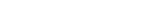MathExamle.com# Multiplication and Division of integers and decimals

Create worksheets of mathematical examples with multiplication and division of integers and decimals, select type of a generator and viewing some samples of task

Creates examples with multiplication and division of decimals

5 Variants 4 Levels
 (75×0.088)÷5.5= 18.81÷13.75= 9.6÷1.28= (1.536÷38.4)÷1.25=
Variant: A Level: Difficult

Creates examples with a division operation of decimals by a column method

1 Variant 3 Levels
 3.3 0.6
 15.4 7
 19.8 1.8
 0.3 0.2
Variant: 1 Level: Easy

Creates examples with a division operation of integers, the answers may contain a decimal point

1 Variant 3 Levels
 60 8
 15 5
 48 6
 27 2
Variant: 1 Level: Easy

Creates examples with a long division of integers according to the British system, the answers may contain a decimal point

1 Variant 3 Levels
 40÷5= 5÷50= 92÷4= 54÷50= 54÷25= 88÷25=
Variant: 1 Level: Normal

Creates examples with a long division of decimals according to the British system

1 Variant 3 Levels
 4.8 60
 0.4 23.4
 8.8 35.2
 7 15.4
Variant: 1 Level: Easy